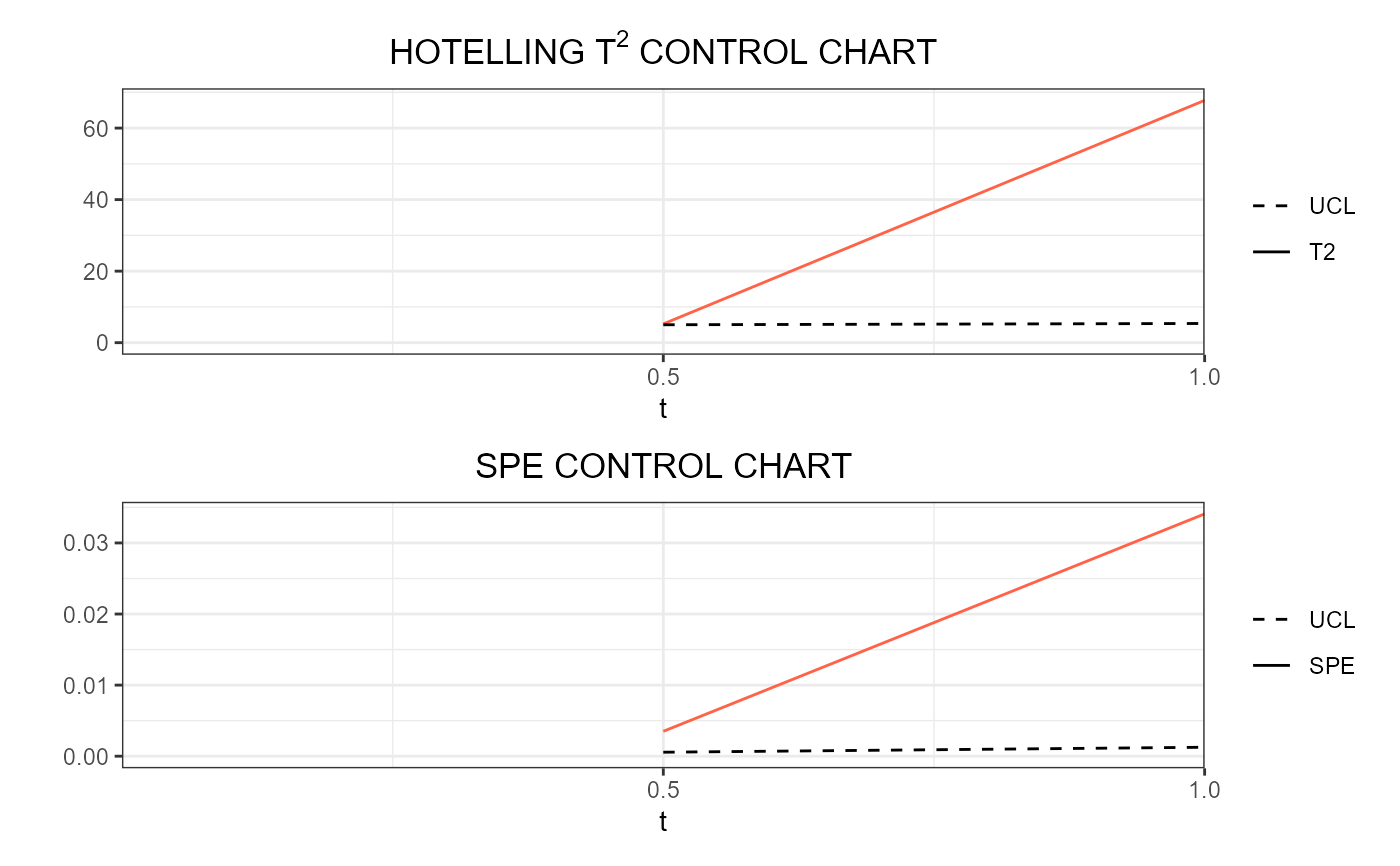This function produces a list of data frames, each of them is produced by regr_cc_fof and is needed to plot control charts for monitoring in real time a functional quality characteristic adjusted for by the effect of multivariate functional covariates.

regr_cc_fof_real_time(
mod_list,
mfdobj_y_new_list,
mfdobj_x_new_list,
mfdobj_y_tuning_list = NULL,
mfdobj_x_tuning_list = NULL,
alpha = 0.05,
include_covariates = FALSE,
absolute_error = FALSE,
ncores = 1
)

## Arguments

mod_list

A list of lists produced by fof_pc_real_time, containing a list of function-on-function linear regression models estimated on functional data each evolving up to an intermediate domain point.

mfdobj_y_new_list

A list created using get_mfd_df_real_time or get_mfd_list_real_time, denoting a list of functional data objects in the phase II monitoring data set, each evolving up to an intermediate domain point, with observations of the functional response variable The length of this list and mod_list must be equal, and their elements in the same position in the list must correspond to the same intermediate domain point.

mfdobj_x_new_list

A list created using get_mfd_df_real_time or get_mfd_list_real_time, denoting a list of functional data objects in the phase II monitoring data set, each evolving up to an intermediate domain point, with observations of the multivariate functional covariates. The length of this list and mod_list must be equal, and their elements in the same position in the list must correspond to the same intermediate domain point.

mfdobj_y_tuning_list

A list created using get_mfd_df_real_time or get_mfd_list_real_time, denoting a list of functional data objects in the tuning data set (used to estimate control chart limits), each evolving up to an intermediate domain point, with observations of the functional response variable. The length of this list and mod_list must be equal, and their elements in the same position in the list must correspond to the same intermediate domain point. If NULL, the training data, i.e. the functional response in mod_list, is also used as the tuning data set. Default is NULL.

mfdobj_x_tuning_list

A list created using get_mfd_df_real_time or get_mfd_list_real_time, denoting a list of functional data objects in the tuning data set (used to estimate control chart limits), each evolving up to an intermediate domain point, with observations of the multivariate functional covariates. The length of this list and mod_list must be equal, and their elements in the same position in the list must correspond to the same intermediate domain point. If NULL, the training data, i.e. the functional covariates in mod_list, are also used as the tuning data set. Default is NULL.

alpha

See regr_cc_fof.

include_covariates

See regr_cc_fof.

absolute_error

See regr_cc_fof.

ncores

If you want parallelization, give the number of cores/threads to be used when creating objects separately for different instants.

## Value

A list of data.frames each produced by regr_cc_fof, corresponding to a given instant.

fof_pc_real_time, regr_cc_fof

## Examples

library(funcharts)
data("air")
air1 <- lapply(air, function(x) x[1:8, , drop = FALSE])
air2 <- lapply(air, function(x) x[9:10, , drop = FALSE])
mfdobj_x1_list <- get_mfd_list_real_time(air1[c("CO", "temperature")],
n_basis = 15,
lambda = 1e-2,
k_seq = c(0.5, 1))
mfdobj_x2_list <- get_mfd_list_real_time(air2[c("CO", "temperature")],
n_basis = 15,
lambda = 1e-2,
k_seq = c(0.5, 1))
mfdobj_y1_list <- get_mfd_list_real_time(air1["NO2"],
n_basis = 15,
lambda = 1e-2,
k_seq = c(0.5, 1))
mfdobj_y2_list <- get_mfd_list_real_time(air2["NO2"],
n_basis = 15,
lambda = 1e-2,
k_seq = c(0.5, 1))
mod_list <- fof_pc_real_time(mfdobj_y1_list, mfdobj_x1_list)
cclist <- regr_cc_fof_real_time(
mod_list = mod_list,
mfdobj_y_new_list = mfdobj_y2_list,
mfdobj_x_new_list = mfdobj_x2_list)
plot_control_charts_real_time(cclist, 1)